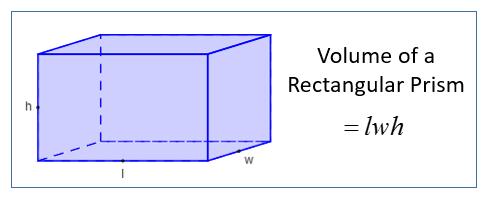# JSN-SR04T converting cm to litres

Hi
I am new to Arduino and I am using a jsn-sr04t with a Uno to read measurements in cm in a rectangular
water tank using the NewPing sketch. I would like to convert the cm values to litres. I know the volume of the tank and litres per cm, but I cannot find any code to convert to litres. Any help would be appreciated.

Andypc

All you need to know is the volume and the highest level and distance to the bottom. It's a simple linear relation between the two.

I cannot find any code to convert to litres.

Forget the code for now. You say that you know the number of litres per cm so let's suppose that it is 2 litres per centimetre and I told you that the level had risen 3 centimetres. How many litres have been added to the tank ?

it is a school math:Does the sketch contain this line?
Serial.print(sonar.ping_cm());

Do you know how many liters per centimeter tank?
e.g. 12.345 litres per cm.

Declare a variable at the top of the sketch.
float tankVolume; // holds volume in litres

Then calculate in the sketch..
tankVolume = sonar.ping_cm() * 12,345; // calculated volume

Then print that tankVolume.
Serial.print(tankVolume);
Serial.println(" litres");
Leo..

Hi
Many thanks for your replies. I did not have a problem with the maths, just what the code was to put into the sketch.

Regards Andy

andypc:
Hi
Many thanks for your replies. I did not have a problem with the maths, just what the code was to put into the sketch.

Regards Andy

Declare a const variable with a sensible name to the number of litres per cm
Declare a const variable with a sensible name for the distance in cm from the sensor to the bottom of the tank
Read the distance from the sensor to the water in the tank and put the answer in a variable
Do the maths using the data that you have

``````byte litresOfWater = (distanceToBottom - distanceToWater) * litresPerCm
``````